# Congruent Angles

In geometry, an angle is formed when two rays are joined together at a common point. The common point here is called node or vertex, and the two rays are called arms of the angle. The angle is represented by the symbol ‘∠’. The word angle came from the Latin word “Angulus”.

In other words, an angle is a form of geometrical shape that is constructed by joining two rays to each other at their end-points. The angle can also be represented by three letters of the shape that define the angle, with the middle letter being where the angle is (i.e.its vertex). Angles are generally named using Greek letters such as θ, α, β, etc. However, the angles can be classified into different types based on their measures. In this article, you will learn the congruent angles definition, examples of congruent angles along with construction.

Before getting into congruent angles, let’s understand the term “congruent”. In geometry, congruent means that one figure is identical to another in shape and size. That means, when we place one figure on the other, they will be superimposed exactly. These figures could be either line segment, polygon, angle, or 3D shape. Corresponding angles on congruent figures are always congruent.

## Congruent Angles Meaning

Congruent angles are two or more angles that are identical to each other. Thus, the measure of these angles is equal to each other. The type of angles does not make any difference in the congruence of angles, which means they can be acute, obtuse, exterior, or interior angles.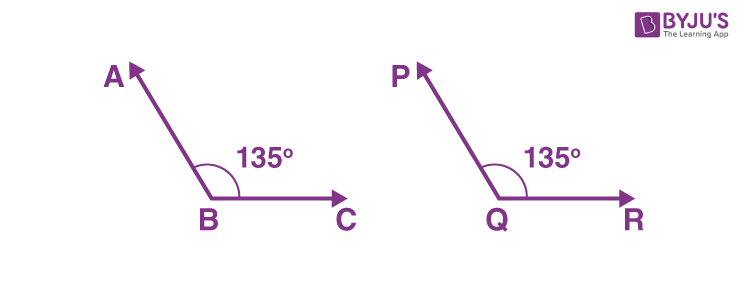In the figure given above, ∠ABC ≅ ∠PQR and is read as “The angle ABC is congruent to the angle PQR”.

### Congruent Angles Symbol

We know that the symbol of congruence in geometry is ““. If ∠A and ∠B have the same measure, then they are said to be equal or congruent. That means ∠A is congruent to ∠B and ∠A = ∠B or ∠A ∠B.

### Congruent Angles Rules

Below are the list of rules for congruence angles.

• The only condition for two angles to be congruent is that the measures of angle measure are the same.
• The length and direction of the two arms making up these congruent angles are irrelevant.

### Congruent Angles Construction

It is possible to construct an angle congruent to a given angle with a compass and ruler. It is just to copy the angle construction.

One of the easiest ways to construct congruent angles is to draw two parallel lines cut by a transversal. And we know that the corresponding angles are congruent in this case.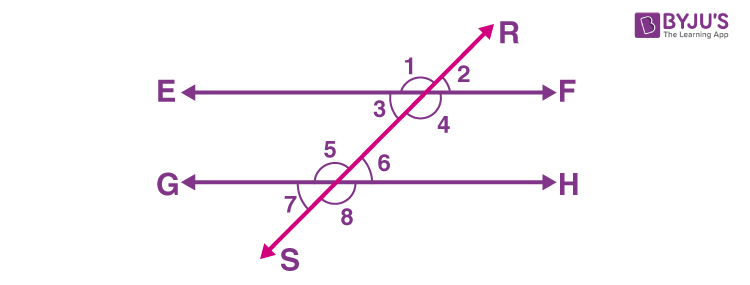In the above figure, RS is the transversal made by two parallel lines EF and GH. Here, the pairs of corresponding angles are congruent. Thus, the pair of congruent angles are:

∠1 and ∠5

∠2 and ∠6

∠3 and ∠7

∠4 and ∠8

Apart from these pairs, we can also write other sets of angles such as alternate angles that are the congruent angles in parallel lines EF and GH

## Congruent Angles Examples

Below are some examples of congruent angles.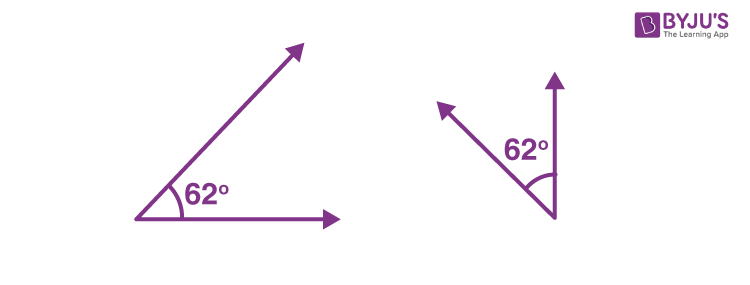The above figure shows the congruent angles of measure of 62 degrees even though they have unequal arms (arms do not have equal length).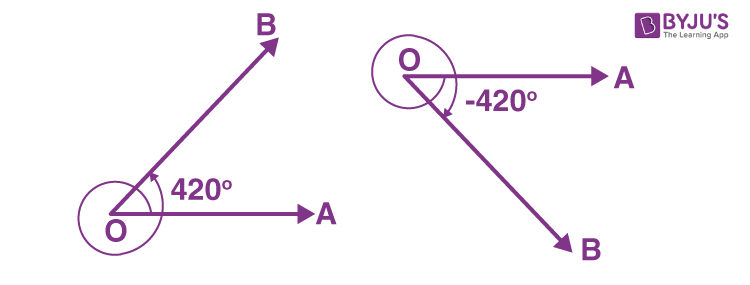In the above figure, the direction of terminals by which the angles made are different yet they are said to be congruent.

### How to find Congruent Angles

Let us consider the figure below to understand how to find the congruent angles.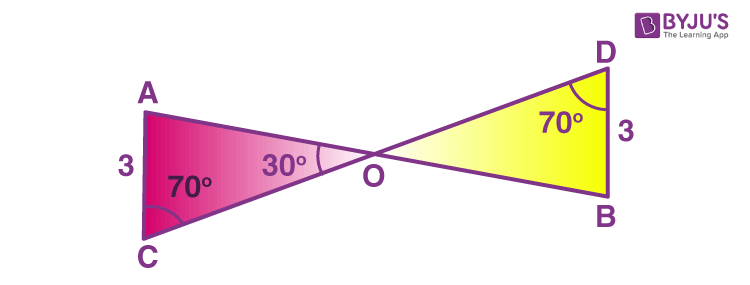Here, ∠OCA and ∠ODB have equal measure of angles so they are called congruent.

Visit www.byjus.com to get more information about different angles and their properties. Also, download BYJU’S – The Learning App to access interactive videos of maths and science concepts.

## Frequently Asked Questions on Congruent Angles

### What are congruent angles?

The angles which have the same measure are called congruent angles.

### How do you find congruent angles?

Congruent angles can be found just by observing the measure of the given angles. However, the length and direction of terminals by which these angles are made need not be the same.

### Do congruent angles add up to 180?

We know that the measure of congruent angles is the same and it is not necessary that they add up to 180 degrees.

### Which pairs of angles are congruent?

When a transversal is drawn through two lines (may be parallel or non-parallel), then the following pairs of angles are congruent.
Interior angles
Exterior angles
Pairs of corresponding angles
Pairs of alternate interior angles
Pairs of alternate exterior angles
Pairs of interior angles on the same side of the transversal

### Are the same side interior angles congruent?

Yes, the pairs of interior angles on the same side of the transversal are congruent.

### What is the symbol for congruent angles?

If ∠X and ∠Y have the same measure, then they are said to be equal or congruent. That means ∠X is congruent to ∠Y and ∠X = ∠Y or ∠X ≅ ∠Y.